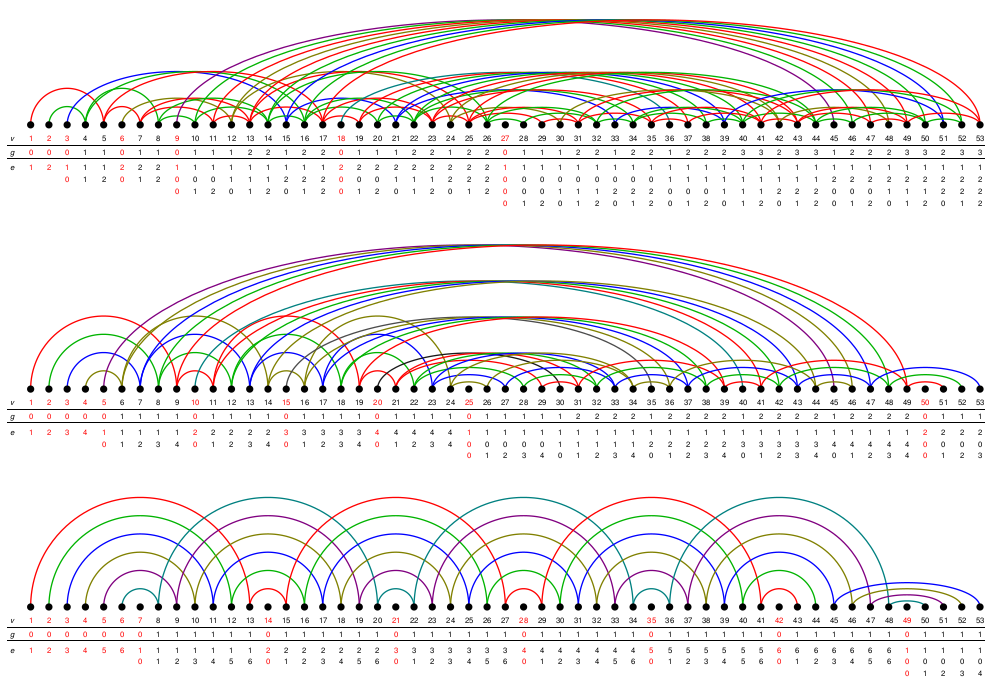## Data

• Title: The center of $$\mathrm{SL}_{2}$$ tilting modules
• Authors: Daniel Tubbenhauer and Paul Wedrich
• Status: Glasg. Math. J. 64 (2022), no. 1, 165-184. Last update: Mon, 25 Jan 2021 09:24:25 UTC
• ArXiv version = 0.99 published version
• LaTex Beamer presentation: Slides, Slides2, Slides3

## Abstract

In this note we compute the centers of the categories of tilting modules for $$G=\mathrm{SL}_{2}$$ in prime characteristic, of tilting modules for the corresponding quantum group at a complex root of unity, and of projective $$G_{g}T$$-modules when g=1,2.

## A few extra words

Let $$\mathbb{K}$$ denote an algebraically closed field and $$\mathbf{Tilt}=\mathbf{Tilt}(\mathrm{SL}_{2})$$ the additive $$\mathbb{K}$$-linear category of (left-)tilting modules for the algebraic group $$\mathrm{SL}_{2}$$. In this note we compute the (categorical) center $$\mathbf{Z}(\mathbf{Tilt})$$ of $$\mathbf{Tilt}$$, using the explicit description of the Ringel dual of $$\mathrm{SL}_{2}$$.
These Ringel duals are quivers living on certain infinite and fractral graphs, and are basically fractal-zigzag-type algebras on these graphs:The full subquivers containing the first 53 vertices of the quiver underlying $$\mathrm{Z}_{\mathsf{p}}$$ for $$\mathsf{p}\in\{3,5,7\}$$, showing from top to bottom the numbers v of the vertices, the generation of v (the number of non-zero digits of v in its $$\mathsf{p}$$-adic expansion minus one) and the $$\mathsf{p}$$-adic expansion of v.
The main theorem then is that we have an (explicit) isomorphism of $$\mathbb{K}$$-algebras between $$\mathbb{K}[X_{v}\mid v\in\mathbb{N}]/\langle X_{v}X_{w}\mid v,w\in\mathbb{N}\rangle$$ and $$\mathbf{Z}(\mathbf{Tilt})$$.
Finally, we also compute the centers for the category of tilting modules in the quantum group case (which is generation 1) as well as for projective $$G_{g}T$$-modules for g=1,2 (generations 1 and 2), both for $$\mathrm{SL}_{2}$$. In these cases the results are similar as for the algebraic group, but much easier and can be thought off as an approximation of order 1 or 2 to the group case.